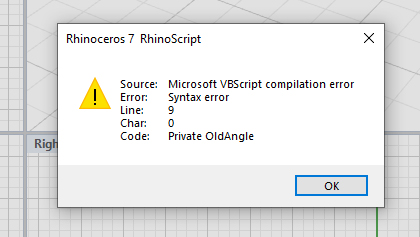# ConeByAngle .rvb by Pascal error in V7 what is needed?

Hi,
V7
I am making buttons for scripts etc I have.

I see I have ConeByAngle.rvb by Pascal.
@pascal
What changes if any are needed for V7 , I click button and get error , it was for V4, what is needed now ?

Hi Steve - Here is a quick and dirty script that should work OK in
V4…To use the script, extract and save the .rvb file from the attached
zip archive, then drag and drop the saved rvb over an open Rhino V4 or
v5 window. This will load the script, set it up to load on startup in
the future and register the alias

ConeByAngle

that will run the script much like a regular command. An alias can be
typed or added to a toolbar button or keyboard shortcut (F-key).

It assumes the angle you want to specify is the one between the straight
edge of the cone and a radius of the base circle…

It is crude- the direction constraint is set to None so that ‘non
Cplanar’ input can be correctly used. It should not be hard to make this
better, but I’d prefer to do it using V5 compatible scripting.

Pascal Golay

here is the code:-
Option Explicit
'Script written by Pascal
’ RMA
'Script version Thursday, March 21, 2013

Private OldAngle
If isEmpty(OldAngle) Then
OldAngle = 60
End If

'Call ConeByAngle()

Sub ConeByAngle()

``````Dim Ang, rad, apts

Dim input(1)
ang = Rhino.GetReal("Set the angle of the cone side.", OldAngle, 0.1, 99.9)
If isNull(input(0)) Then Exit Sub
OldAngle = ang

aPts = Rhino.GetPoints(True,, "Set center of the base.", "Set radius.", 2)
If Not isArray(aPts) Then Exit Sub
If Ubound(aPts) < 1 Then Exit Sub
Rhino.Command "_Cone _DirectionConstraint=_None W" & Rhino.Pt2Str(aPts(0),, True) & "W" & Rhino.Pt2Str(aPts(1),, True) & H
``````

End Sub

I had got as far as:-
Bold the added coding for button use, Italic the deleted coding for button use.

-_Runscript (

Option Explicit

'Script written by Pascal

’ RMA

'Script version Thursday, March 21, 2013

Call Main()

Sub Main()

Private OldAngle

If isEmpty(OldAngle) Then

OldAngle = 60

End If

'Call ConeByAngle()

Sub ConeByAngle()

Dim input(1)

ang = Rhino.GetReal(“Set the angle of the cone side.”, OldAngle, 0.1, 99.9)

If isNull(input(0)) Then Exit Sub

OldAngle = ang

aPts = Rhino.GetPoints(True, “Set center of the base.”, “Set radius.”, 2)

If Not isArray(aPts) Then Exit Sub

If Ubound(aPts) < 1 Then Exit Sub

Rhino.Command “_Cone _DirectionConstraint=_None W” & Rhino.Pt2Str(aPts(0), True) & “W” & Rhino.Pt2Str(aPts(1), True) & H

End Sub
)

So how did I do ?

Steve

Hello- it would help to know what the error message is.

-Pascal

Hi,
V7